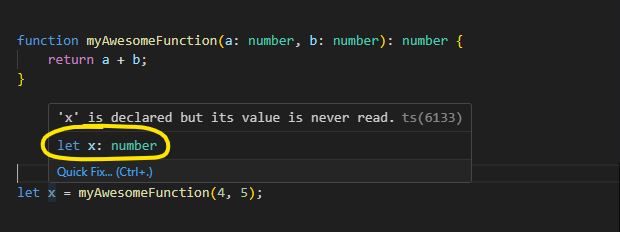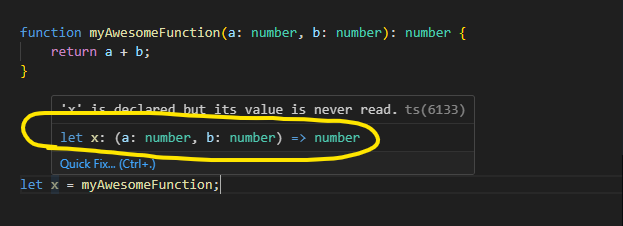# First Class Functions in JavascriptCan you spot the difference between these two lines of code...

```const resultA = myFunction();
const resultB = myFunction;```

The first line is assigning a variable to the result of a function, the second line is assigning a variable to the function itself.

## Introducing First Class Functions

A programming language is said to have first class functions when it treats functions as first class citizens. In simpler terms, it means a function in such a language can be:

1. Passed as an argument to another function.
2. Returned as a value from another function.
3. Assigned to a variable.

Languages like JavaScript, TypeScript, and many others support this powerful concept.

### Demonstrating First Class Functions with TypeScript

Consider the following example written in TypeScript:

`function myAwesomeFunction(a: number, b: number): number {    return a + b;}let x = myAwesomeFunction(4, 5);`

In the above code, we declare a simple function `myAwesomeFunction` which takes two numbers and returns their sum. We can then execute this function by adding brackets after its name. By doing so, the sum of 4 and 5 is assigned to the variable x.However, the magic of first class functions allows us to assign the function itself to a variable, like this:

`let x = myAwesomeFunction;`

Now, x refers to the function rather than the result of the function. When you hover over x in an IDE like VSCode, it would show that x is a function with a particular TypeScript function signature.By assigning the function to a variable, you gain the flexibility to pass it around, assign it to another variable, or even execute it just like the original function. This is depicted as:

`x(4, 5);  // This will return 9, the sum of 4 and 5`

## Practical Applications

Such flexibility opens doors to more abstract coding patterns. Imagine having a code snippet that filters an array based on a certain condition. This condition, being a function, can be swapped out with another, allowing you to reuse the filtering mechanism with different criteria.

For instance:

```function isAboveFive(num: number): boolean {    return num > 5;}
function isAboveTen(num: number): boolean {    return num > 10;}
let numbers = [1, 6, 8, 11, 15];let filteringFunction = isAboveFive;let filteredNumbers = numbers.filter(filteringFunction);```

Here, filteringFunction can point to either isAboveFive or isAboveTen, offering dynamic filtering capabilities.

This modular approach can make your code more readable and extendable, and it beautifully showcases the power of treating functions like any other variable.

## In Practise

First class functions can introduce a new dimension of dynamism and modularization to your code. If you've got creative applications of this concept or ways in which you've made your code more intuitive and maintainable using it, do share!

Understanding and mastering first class functions is a stepping stone towards writing cleaner and more maintainable code. It adds another tool to your developer toolbox, helping you craft efficient solutions and embrace best coding practices.